Worksheets and No Prep Teaching Resources Math Worksheets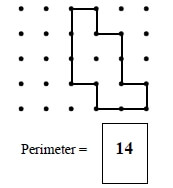Perimeter Activities, Worksheets, Printables, and Lesson Plans

Fewer Problems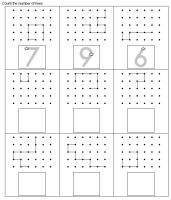Line length of 3 to 9 - first row answers given Line length of 3 to 9 Line length of 10 to 19 - first row answers given Line length of 10 to 19

More Problems Per Page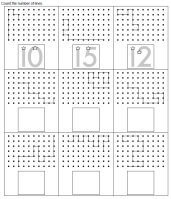Line length of 3 to 9 - first row answers given Line length of 3 to 9 Line length of 10 to 19 - first row answers given Line length of 10 to 19

Connect the Line from A to B - Draw the Path to the Given Line Length (Grades 3-5)
Easier - Starting and Ending Part of Line Given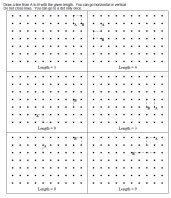Draw the line length of 3 to 9 - first row answers given Draw the line length of 3 to 9 Draw the line length of 10 to 19 - first row answers given Draw the line length of 10 to 19

Draw the Line from A to B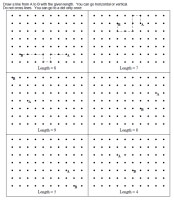Draw the line length of 3 to 9 - first row answers given Draw the line length of 3 to 9 Draw the line length of 10 to 19 - first row answers given Draw the line length of 10 to 19

Easier - Dot Grid Shown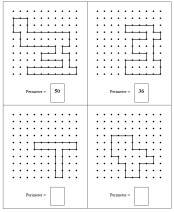Write the Perimeter - first row answers given (smaller shapes) Write the Perimeter - first row answers given (larger shapes) Write the Perimeter (smaller shapes) Write the Perimeter (larger shapes)

Rectangles Shown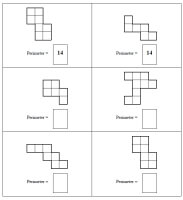Write the Perimeter - first row answers given (smaller shapes) Write the Perimeter - first row answers given (larger shapes) Write the Perimeter (smaller shapes) Write the Perimeter (larger shapes)

More Challenging - Indent Lines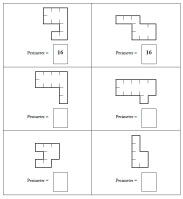Write the Perimeter - first row answers given (smaller shapes) Write the Perimeter - first row answers given (larger shapes) Write the Perimeter (smaller shapes) Write the Perimeter (larger shapes)

Most Challenging - Indent Lines (fewer indent lines given)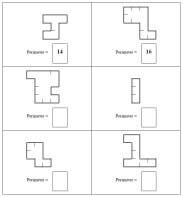Write the Perimeter - first row answers given (smaller shapes) Write the Perimeter - first row answers given (larger shapes) Write the Perimeter (smaller shapes) Write the Perimeter (larger shapes)

Find the perimeter of each figure
Find the perimeter of each figure using a ruler
Perimeter: Find the value of n
Find the area of each figure
Find the value of n
Find the area and perimeter of each rectangle (no graphics)
Find the perimeter of a complex polygon (composed of 2 rectangles)
Find the perimeter of a complex polygon (composed of 3 rectangles)
Find the perimeter of a complex polygon (composed of 4 rectangles)
Find the area and perimeter of a complex polygon (composed of 2 rectangles)
Find the area and perimeter of a complex polygon (composed of 3 rectangles)
Find the area and perimeter of a complex polygon (composed of 4 rectangles)
Area and Perimeter Mixed Review

Find the perimeter of each figure
Find the perimeter of each figure using a ruler
Perimeter: Find the value of n
Find the area of each figure
Find the value of n
Find the area and perimeter of each rectangle (no graphics)
Find the perimeter of a complex polygon (composed of 2 rectangles)
Find the perimeter of a complex polygon (composed of 3 rectangles)
Find the perimeter of a complex polygon (composed of 4 rectangles)
Find the area and perimeter of a complex polygon (composed of 2 rectangles)
Find the area and perimeter of a complex polygon (composed of 3 rectangles)
Find the area and perimeter of a complex polygon (composed of 4 rectangles)
Area of a parallelogram (lengths are whole numbers)
Area of a parallelogram (lengths are decimals)
Area of a parallelogram (lengths are fractions)
Area of a triangle (lengths are whole numbers)
Area of a triangle (lengths are decimals)
Area of a triangle (lengths are fractions)
Find the circumference (graphics; lengths are whole numbers)
Find the circumference (graphics; lengths are decimals)
Find the circumference (graphics; lengths are fractions)
Find the circumference (lengths are whole numbers)
Find the circumference (lengths are decimals)
Find the circumference (lengths are fractions)
Area and Perimeter Mixed Review (Grade 5)

Find the perimeter of each figure
Find the perimeter of each figure using a ruler
Perimeter: Find the value of n
Find the area of each figure
Find the value of n
Find the area and perimeter of each rectangle (no graphics)
Find the perimeter of a complex polygon (composed of 2 rectangles)
Find the perimeter of a complex polygon (composed of 3 rectangles)
Find the perimeter of a complex polygon (composed of 4 rectangles)
Find the area and perimeter of a complex polygon (composed of 2 rectangles)
Find the area and perimeter of a complex polygon (composed of 3 rectangles)
Find the area and perimeter of a complex polygon (composed of 4 rectangles)
Area and Perimeter Mixed Review (Grade 4)

Part 2
Area of a parallelogram (lengths are whole numbers)
Area of a parallelogram (lengths are decimals)
Area of a parallelogram (lengths are fractions)
Area of a triangle (lengths are whole numbers)
Area of a triangle (lengths are decimals)
Area of a triangle (lengths are fractions)
Find the circumference (graphics; lengths are whole numbers)
Find the circumference (graphics; lengths are decimals)
Find the circumference (graphics; lengths are fractions)
Find the circumference (lengths are whole numbers)
Find the circumference (lengths are decimals)
Find the circumference (lengths are fractions)
Area and Perimeter Mixed Review (Grade 5)

Perimeter, Circumference, and Area
Find area of triangles (given coordinates)
Find area of rectangles and squares (given coordinates)
Find area of trapezoids (given coordinates)
Find area review (given coordinates)
Area and perimeter word problems: rectangles and parallelograms
Area and perimeter: rectangles with fractions
Area and perimeter of rectangles (algebra)
Area and perimeter: triangles
Area and perimeter: trapezoids
Area and perimeter: triangles (with fractions)
Area and perimeter: trapezoids (with fractions)
Pythagorean theorem
Circles: Find the circumference
Circles: Find the area
Circles: Find the circumference and area
Perimeter and circumference - Word Problems Printable
Area - Word Problems Printable
Perimeter, Circumference, and Area Review Printable

Area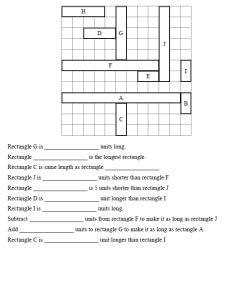Length: With Addition and Subtraction